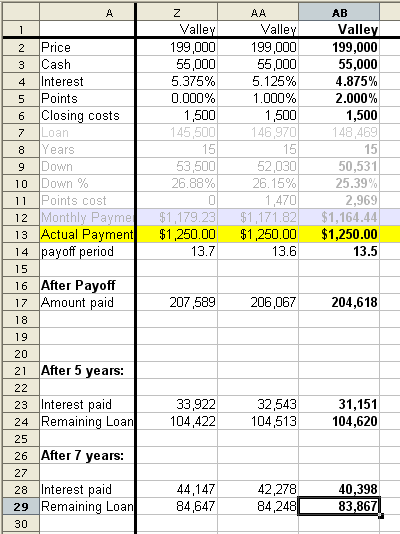# Mortgage Points vs.Rate Calculator Spreadsheet

## What is Mortgage Points vs.Rate Calculator Spreadsheet?This spreadsheet (in Open Office format) calculates payoff period, remaining loan after 5, 7, and 10 years, total interest paid, etc. It includes a set of payoff functions in StarOffice Basic. It is usefull to calculate, among other things, whether you should pay mortgage points up front, or go with a higher mortgage rate.

I was thinking of a way to compare different loans with. I finally came up with this spreadsheet where these items should be entered: The first set is probably constant througout the whole spreadsheet.

• Cost of the real-estate
• Amount of cash available for downpayment
• Monthly payment that you can afford

These parameters you need to enter for each mortgage offer:

• Interest rate
• Number of points
• Closing costs

## Example

The spreadsheet will calculate how large loan you have to take. For example (see the screenshot), I want to buy property that costs \$199,000, I have \$55,000 available for downpayment and closing costs, and I know I can afford to pay \$1,250 every month. Now for each mortgage offer, I enter rate, points, and fees. I decided that I might sell the property after 7 years, so I am looking for an offer that has the lowest "Remaining Loan" after that period (see cell AB29). The offer from the Valley National Bank was the best: 4.875% rate with 2.0 points. So the spreadsheet calculates that I should take the loan of \$148,469 (cell AB7). I will put a downpayment of \$50,531 (cell AB9), and the remainder to \$55,000 will be used to pay bank fees and points.

## Usage

• Enter propery price, cash available, and monthly payments you can afford in cells B2,B3,B13. These will be propagated througout the whole spreadsheet.
• For each mortgage offer, enter the name of the bank, interest, points, and closing costs in each column.
• If you will keep the property until you pay it off, try to minimize "Amount paid" (row 17).
• If you intend to sell after 5 years for example, look for minimal value under "After 5 years/ Remaining Loan".

## Requirements

The implementation of the payment function in StarOffice BASIC is extracted for your convenience in:

## Payment Function

The notation is:

```r  is monthly rate factor(e.g. r = 100% + 4.875%/12 = 1.0040625)
p  is monthly payment    (e.g. \$1,250 per month)
v0 is initial loan       (e.g. \$148,469)
v  is remaining loan after some period (e.g. after 7yrs=84mo, v=\$83,867).
```

When you get a loan, after the first month you incurr interest on the whole loan v0, and make one payment of p.

`balance  = v0*r - p`

The next month you pay the interest on the balance:

```balance_2=   (v0 r - p)r - p
balance_3=  ((v0 r - p)r - p)r - p
balance_4= (((v0 r - p)r - p)r - p)r - p```

... and so on. After N payments, the balance is:

```balance_n= v0*r^n  +  p*r^(n-1) + p*r^(n-2) + ... + p
= v0*r^n  +  p *(r^(n-1) + r^(n-2) + ... + 1)

balance_n= v0*r^n  -  p * (1-r^n)/(1-r)
```

And that is the final formula for the payment function (built in -PMT() function). When the balance goes to zero, n = number of payments needed to pay off the debt. Or solve the function for p = default payment needed in order to pay off the debt in n number of months.

```balance_n        =  v0*r^n  -  p * (1-r^n)/(1-r)
default_payment  = (v0*r^n - v)  * (1-r)/(1-r^n)
payoff_period    = Log( (v + p/(1-r)) / (v0 + p/(1-r)) ) / Log(r)
```

The StarOffice BASIC source code macro calculates these three functions. The functions inputs are the period years, and annual rate.